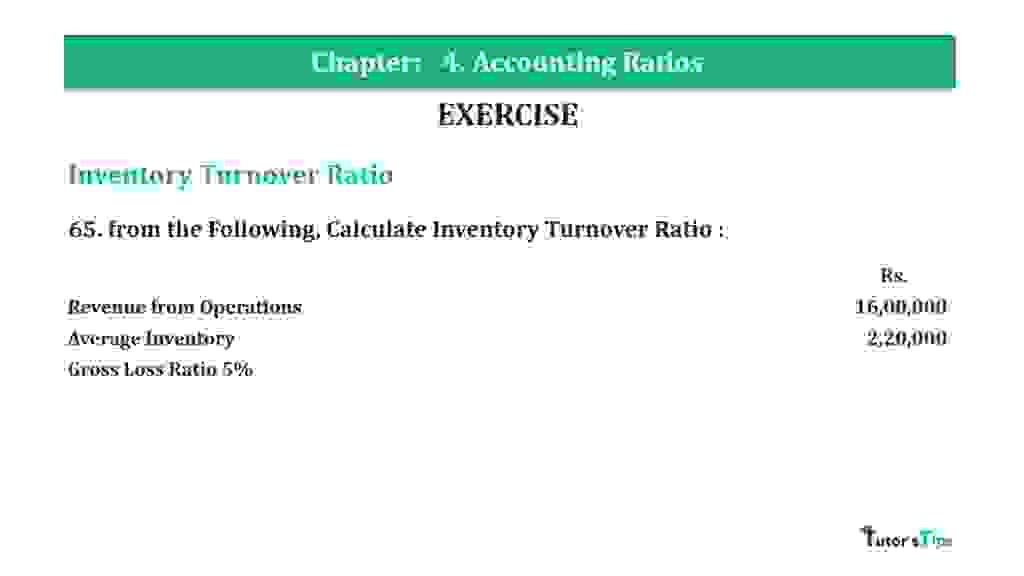# Question 65 Chapter 4 of +2-B – T.S. Grewal 12 ClassQuestion 65 Chapter 4 of +2-B

Interest Coverage Ratio

65. from the Following, Calculate Inventory Turnover Ratio :

 Rs. Revenue from Operations 16,00,000 Average Inventory 2,20,000 Gross Loss Ratio 5%

### The solution of Question 65 Chapter 4 of +2-B: –

 Cost of Revenue from Operations = Revenue from Operations + Gross Loss = Rs. 16,00,000 + Rs. 80,000 = Rs. 16,80,000

 Average Stock = Opening Stock + Closing Stock 2

Average Inventory = Rs. 2,20,000

 Inventory Turnover Ratio = Cost of Goods of Goods Sold Average Stock

 Inventory Turnover Ratio = Rs. 16,80,000 Rs. 2,20,000 = 7.64 Times

Balance Sheet: Meaning, Format & Examples

Thanks, Please Like and share with your friends

Comment if you have any question.

Also, Check out the solved question of previous Chapters: –

### T.S. Grewal’s Double Entry Book Keeping (Vol. II: Accounting for Companies)T.S. Grewal’s Analysis of Financial Statements